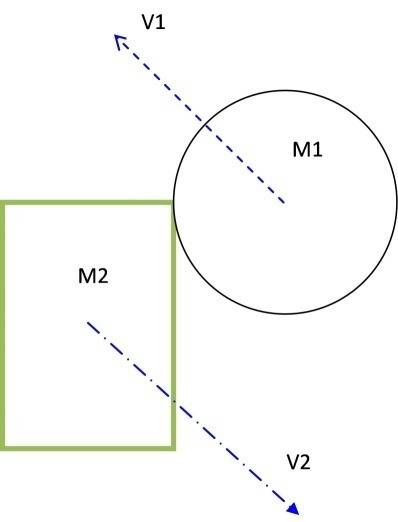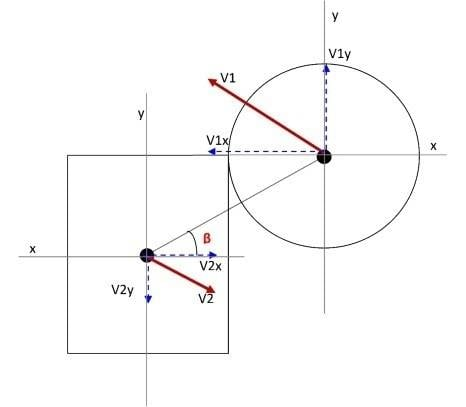# Collision response of two object

knonk
Hi,
how can i find collision response between rectangle and circle like in this picture :i want to identify the direction and magnitude of V1 vector and the V2 vector after they collided , does anyone have some idea about this ?

#### Attachments

Last edited:

Homework Helper
Welcome to PF!

i want to identify the direction and magnitude of V1 vertex and the V2 vertex after they collided , does anyone have some idea about this ?

Hi knonk! Welcome to PF!(your picture doesn't show any vertex marked V1 or V2)

Show us what you've tried, and where you're stuck, and then we'll know how to help!knonk
Okey, in this picture :With :
+ V1 : initial velocity vector of Circle
+ M1 : mass of Circle
+ V2 : initial velocity vector of Rectangle
+ M2 : mass of Rectangle

i want to identify the direction and magnitude of V1 vector and the V2 vector after they collided, does anyone have some idea about this ?

Last edited:
Homework Helper
Hi knonk!(ah, you meant "vector", not "vertex"!)
i want to identify the direction and magnitude of V1 vector and the V2 vector after they collided, does anyone have some idea about this ?

On this forum, the idea is that you do the work, and we give you hints, so …

Show us what you've tried, and where you're stuck, and then we'll know how to help!knonk
Please look at this picture :First, we consider that :
+ v1i : veloc vector of circle BEFORE they collide
+ v2i : veloc vector of rectangle BEFORE they collide
+ v1f : veloc vector of circle AFTER they collide
+ v2f : veloc vector of rectangle AFTER they collide​

How to find V1f and V2f

*Before the collision
+Momentum of the objects:
p1i = m1*v1i
p2i = m2*v2i
Pi = p1i+p2i​
+Kinetic energy of the objects:
ke1i = (1/2)*m1*v1i2
ke2i = (1/2)*m2*v2i2
KEi = ke1i+ke2i​
*After the collision
p1f = m1*v1f
p2f = m2*v2f
Pf = p1f+p2f​
+Kinetic energy of the objects:
ke1f = (1/2)*m1*v1f2
ke2f = (1/2)*m2*v2f2
KEf = ke1f+ke2f​
*Apply the law of the conservation of momentum
Pi = Pf = P
m1*v1i+m2*v2i = m1*v1f+m2*v2f
KEi = Kef
(1/2)*m1*v1i2+(1/2)*m2*v2i2 = (1/2)*m1*v1f2+(1/2)*m2*v2f2​
*Rearranging and combining the two equations above, we get the following:
v1i-v2i = v2f-v1f
V = v1i-v2i
So,
v1f = v2f-V​
*Using the above equation with the equation for P, we get the final results:
v2f = (P+V*m1)/(m1+m2)
v1f = v2f-v1i+v2i
So we know the way how to find V1f and V2f,and we have this :
V1i = V1;
V2i = V2;​
And m1,m2, so we can find v1f,v2f
But seem they have something wrong but i don’t know,
Uhm,Hope u can tell me. Thanks alot.

#### Attachments

Homework Helper
When I see the picture, I feel that both rectangular block and circular block must possesses translational and rotational motion.